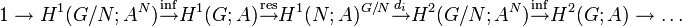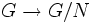# Hochschild-Serre exact sequence

## Definition

This sequence is termed the Hochschild-Serre exact sequence or the inflation-restriction exact sequence.

### General case

Suppose$G$ is a group,$H$ is a normal subgroup of$G$, and$A$ is an abelian group with a$G$-action on it, i.e., a homomorphism$\varphi:G \to \operatorname{Aut}(A)$. We thus have a short exact sequence:$1 \to H \to G \to G/H \to 1$

where$1$ stands for the trivial group.

The inflation-restriction exact sequence or Hochschild-Serre exact sequence is a long exact sequence of cohomology groups given as follows:$1 \to H^1(G/N;A^N) \stackrel{\operatorname{inf}}{\to} H^1(G;A) \stackrel{\operatorname{res}}{\to} H^1(N;A)^{G/N} \stackrel{d_i}{\to} H^2(G/N;A^N) \stackrel{\operatorname{inf}}{\to} H^2(G;A) \to \dots$

Here,$A^N$ denotes the subgroup of$A$ comprising those elements fixed pointwise by every element of$N$. Further:

• The homomorphisms$H^i(G/N;A^N) \stackrel{\operatorname{inf}}{\to} H^i(G;A)$ are inflation homomorphisms corresponding to the quotient map$G \to G/N$.
• The homomorphisms$H^i(G;A) \to H^i(N;A)^{G/N}$ are restriction homomorphisms to$H^i(N;A)$ coupled with the observation that the image is invariant under the natural$G/N$-action induced from the action by conjugation on$N$. PLACEHOLDER FOR INFORMATION TO BE FILLED IN: [SHOW MORE])
• The homomorphisms$H^i(N;A)^{G/N} \to H^{i+1}(G/N;A^N)$ are termed transgression homomorphisms.

### Case of central subgroup

A special case of interest is where$H$ is a central subgroup of$G$. In this case, the$G/N$-action on$H^i(N;A)$ is trivial, and so$H^i(N;A)^{G/N}$ simply becomes$H^i(N;A)$.

### First five terms

The first five terms are important in that they form a five-term exact sequence of significance.$1 \to H^1(G/N;A^N) \stackrel{\operatorname{inf}}{\to} H^1(G;A) \stackrel{\operatorname{res}}{\to} H^1(N;A)^{G/N} \stackrel{d_i}{\to} H^2(G/N;A^N) \stackrel{inf}{\to} H^2(G;A)$